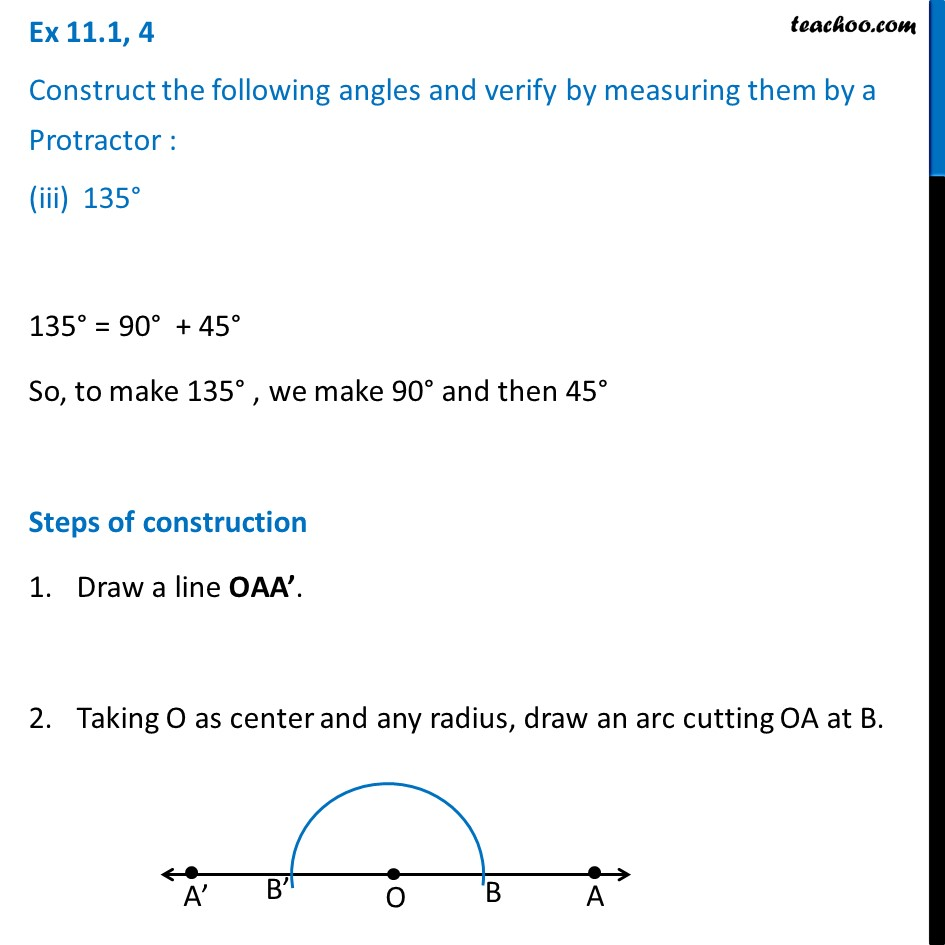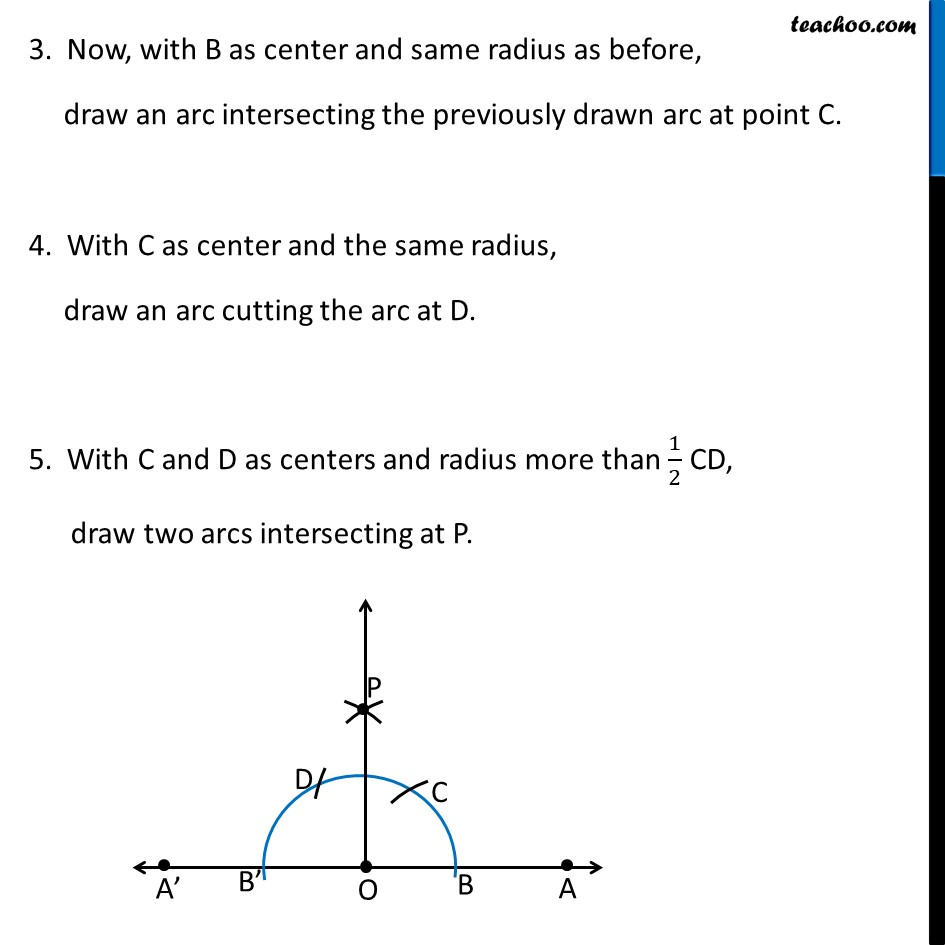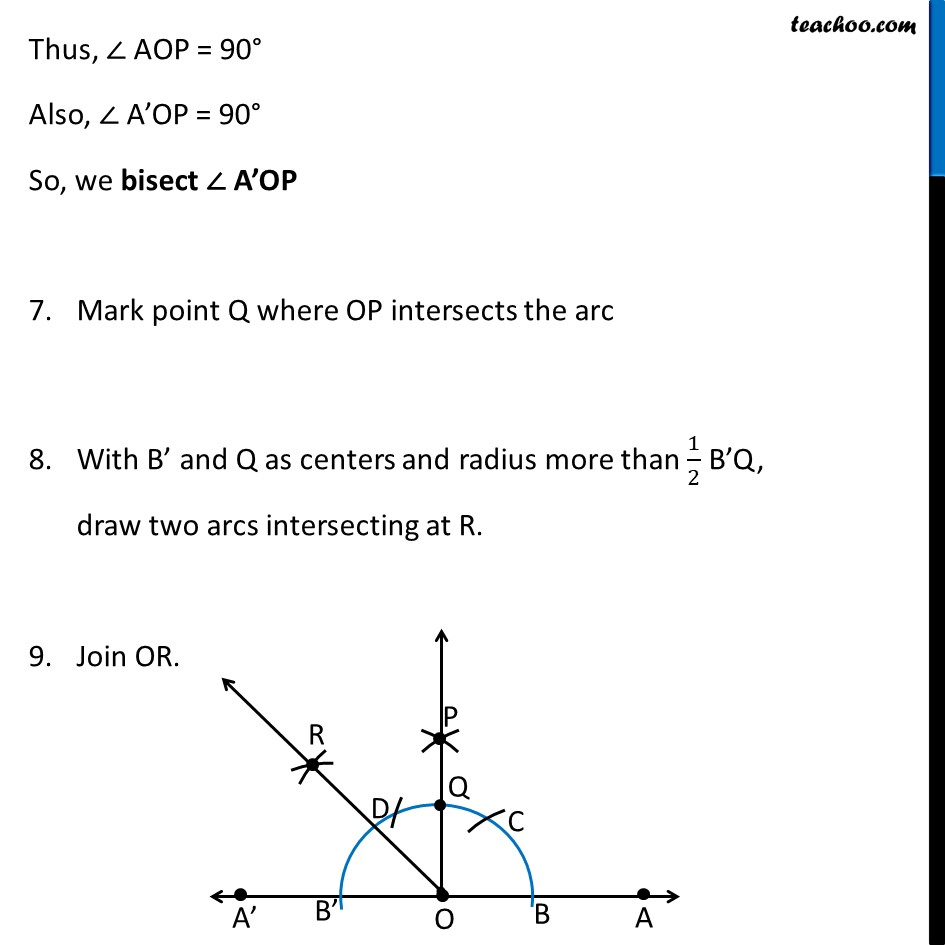1. Chapter 11 Class 9 Constructions
2. Serial order wise
3. Ex 11.1

Transcript

Ex 11.1, 4 Construct the following angles and verify by measuring them by a Protractor : 135° 135° = 90° + 45° So, to make 135° , we make 90° and then 45° Steps of construction Draw a line OAA’. Taking O as center and any radius, draw an arc cutting OA at B. 3. Now, with B as center and same radius as before, draw an arc intersecting the previously drawn arc at point C. 4. With C as center and the same radius, draw an arc cutting the arc at D. 5. With C and D as centers and radius more than 1/2 CD, draw two arcs intersecting at P. Thus, ∠ AOP = 90° Also, ∠ A’OP = 90° So, we bisect ∠ A’OP Mark point Q where OP intersects the arc With B’ and Q as centers and radius more than 1/2 B’Q, draw two arcs intersecting at R. Join OR. ∴ ∠ POR = 45° Thus, ∠ AOR = ∠ AOP + ∠ POR = 90° + 35° = 135° ∴ ∠ AOR = 135°

Ex 11.1